Ex 2.4 (Optional)

Chapter 2 Class 10 Polynomials
Serial order wise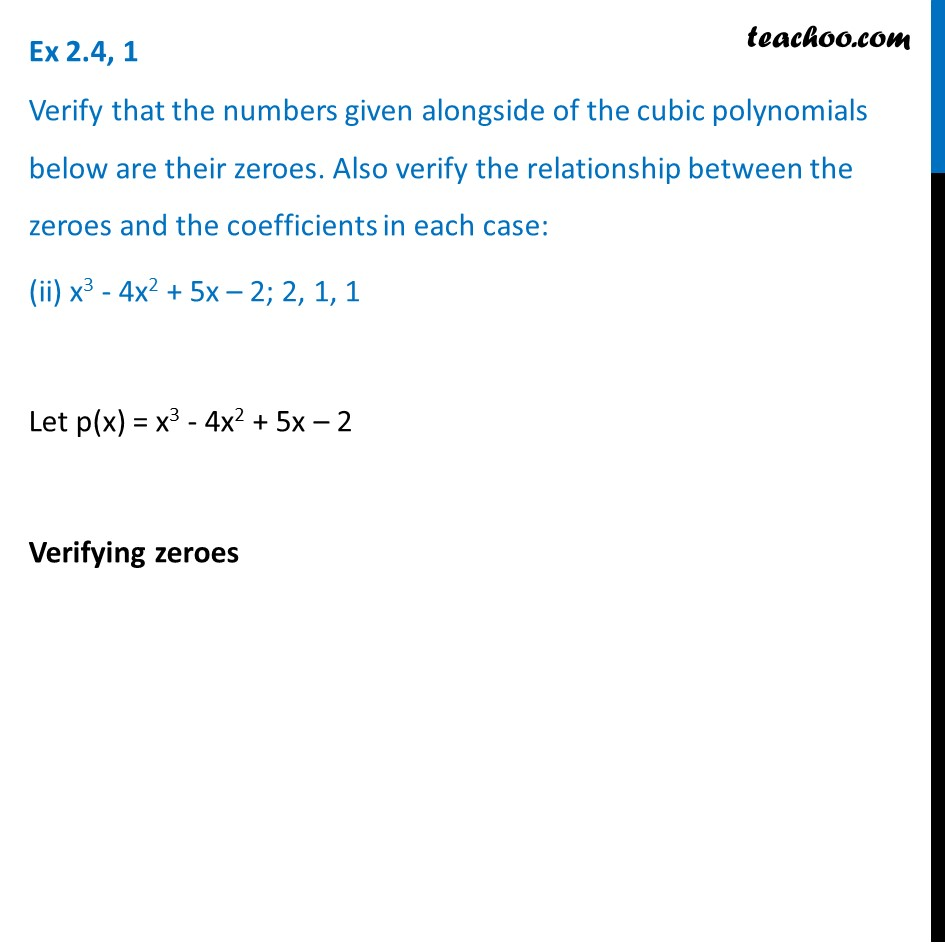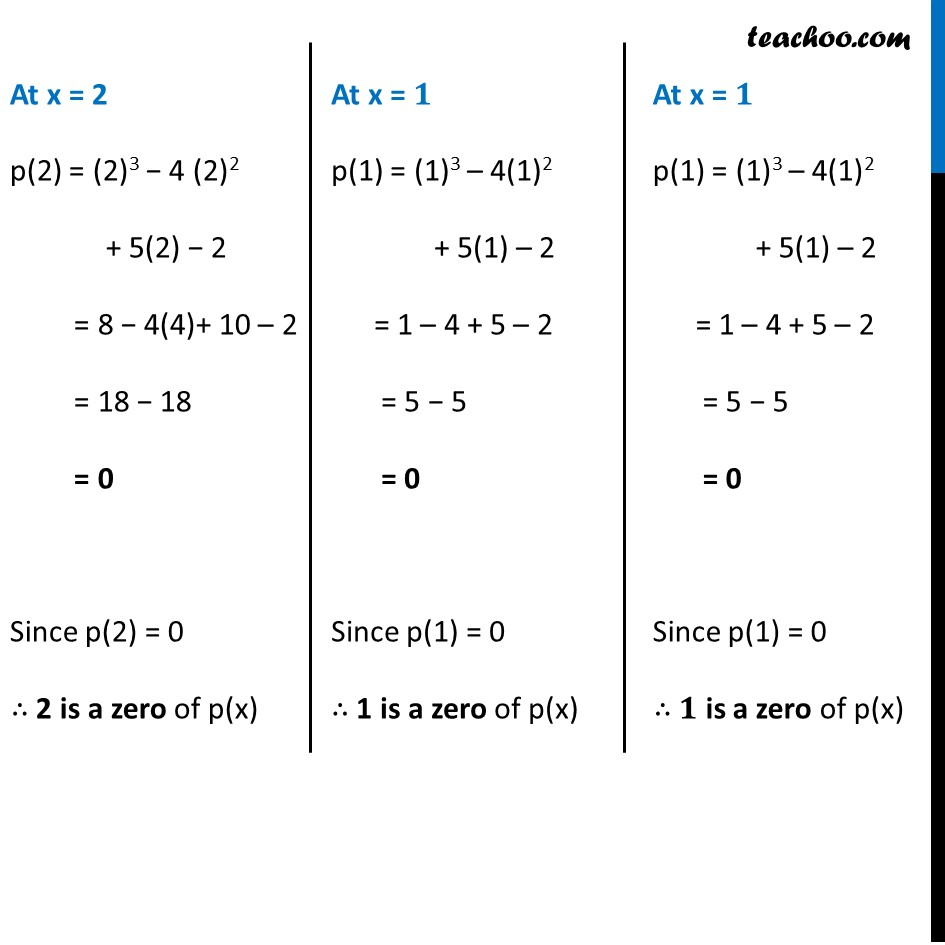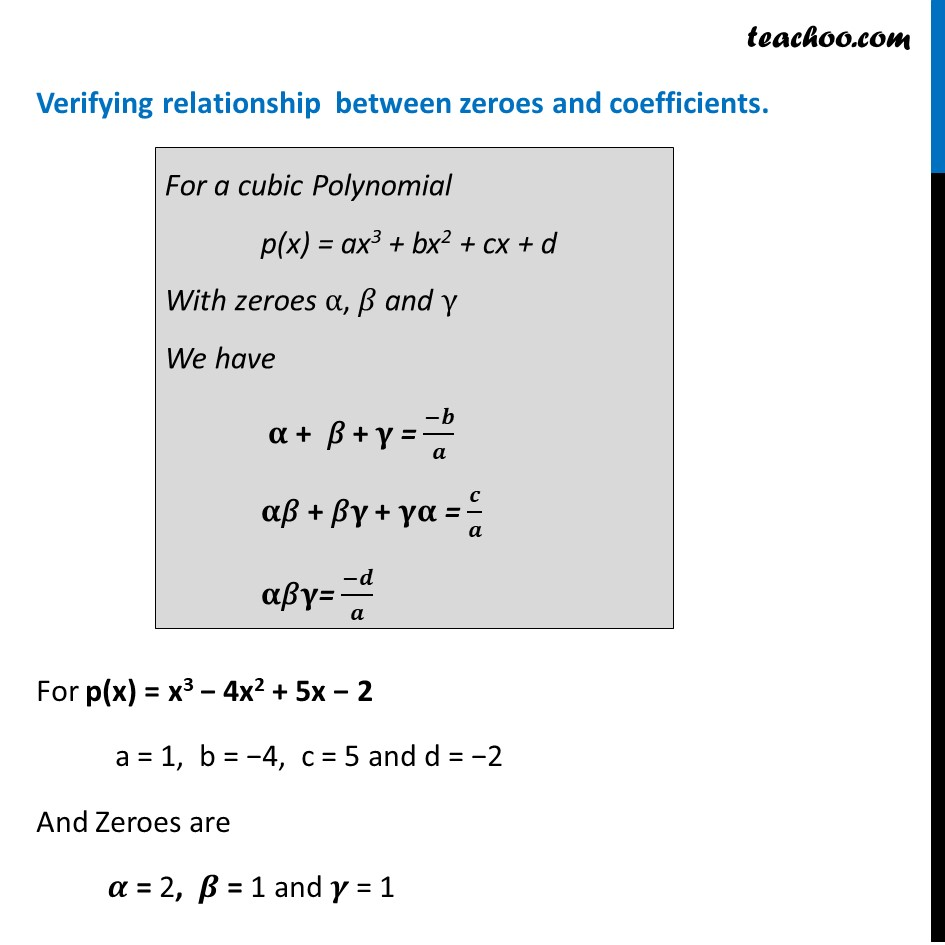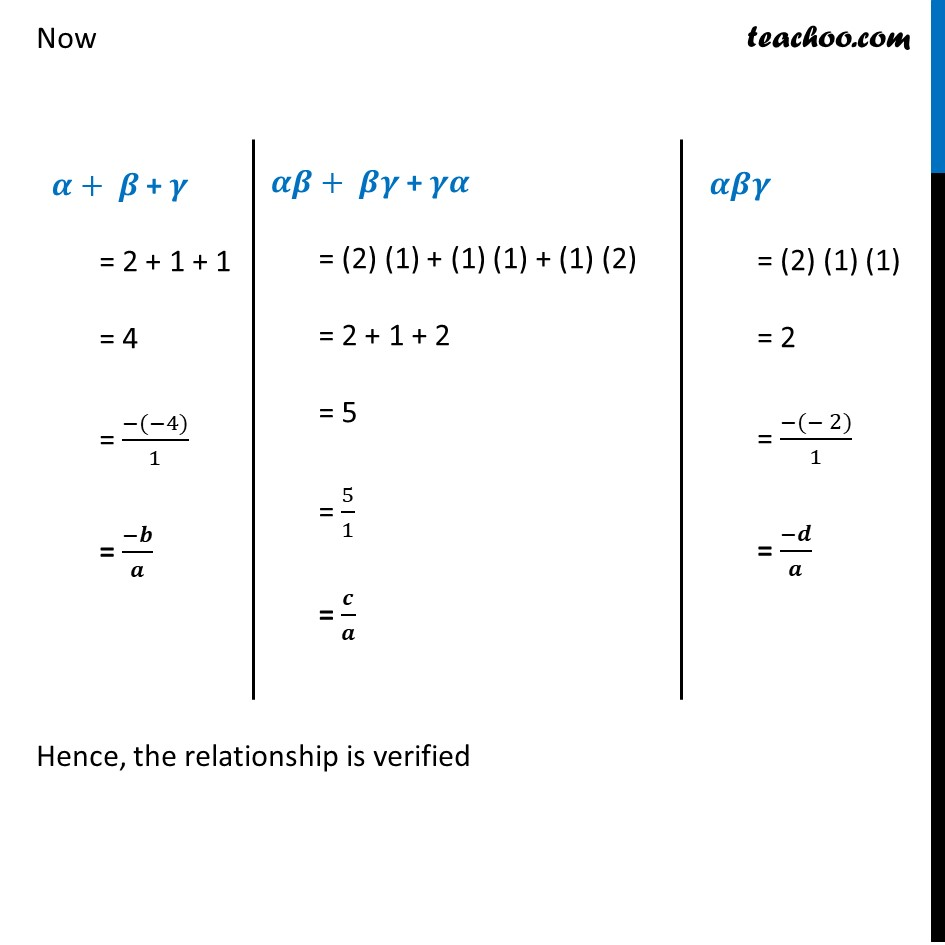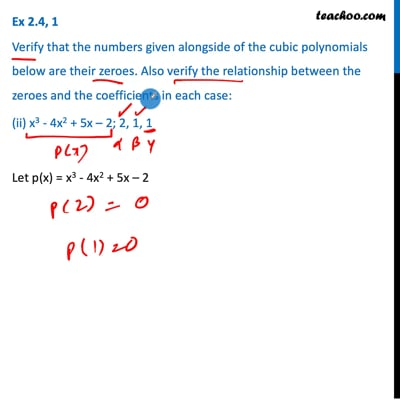This video is only available for Teachoo black users

Introducing your new favourite teacher - Teachoo Black, at only ₹83 per month

### Transcript

Ex 2.4, 1 Verify that the numbers given alongside of the cubic polynomials below are their zeroes. Also verify the relationship between the zeroes and the coefficients in each case: (ii) x3 - 4x2 + 5x – 2; 2, 1, 1 Let p(x) = x3 - 4x2 + 5x – 2 Verifying zeroes At x = 2 p(2) = (2)3 − 4 (2)2 + 5(2) − 2 = 8 − 4(4)+ 10 – 2 = 18 − 18 = 0 Since p(2) = 0 ∴ 2 is a zero of p(x) At x = 𝟏 p(1) = (1)3 – 4(1)2 + 5(1) – 2 = 1 – 4 + 5 – 2 = 5 − 5 = 0 Since p(1) = 0 ∴ 1 is a zero of p(x) At x = 𝟏 p(1) = (1)3 – 4(1)2 + 5(1) – 2 = 1 – 4 + 5 – 2 = 5 − 5 = 0 Since p(1) = 0 ∴ 𝟏 is a zero of p(x) Verifying relationship between zeroes and coefficients. For p(x) = x3 − 4x2 + 5x − 2 a = 1, b = −4, c = 5 and d = −2 And Zeroes are 𝜶 = 2, 𝜷 = 1 and 𝜸 = 1 For a cubic Polynomial p(x) = ax3 + bx2 + cx + d With zeroes α, 𝛽 and γ We have 𝛂 + 𝛽 + 𝛄 = (−𝒃)/𝒂 𝛂"𝛽" + 𝛽𝛄 + 𝛄𝛂 = 𝒄/𝒂 𝛂"𝛽" 𝛄= (−𝒅)/𝒂 Now Hence, the relationship is verified 𝜶+ 𝜷 + 𝜸 = 2 + 1 + 1 = 4 = (−(−4))/1 = (−𝒃)/𝒂 𝜶𝜷+ 𝜷𝜸 + 𝜸𝜶 = (2) (1) + (1) (1) + (1) (2) = 2 + 1 + 2 = 5 = 5/1 = 𝒄/𝒂 𝜶𝜷𝜸 = (2) (1) (1) = 2 = (−(− 2))/1 = (−𝒅)/𝒂